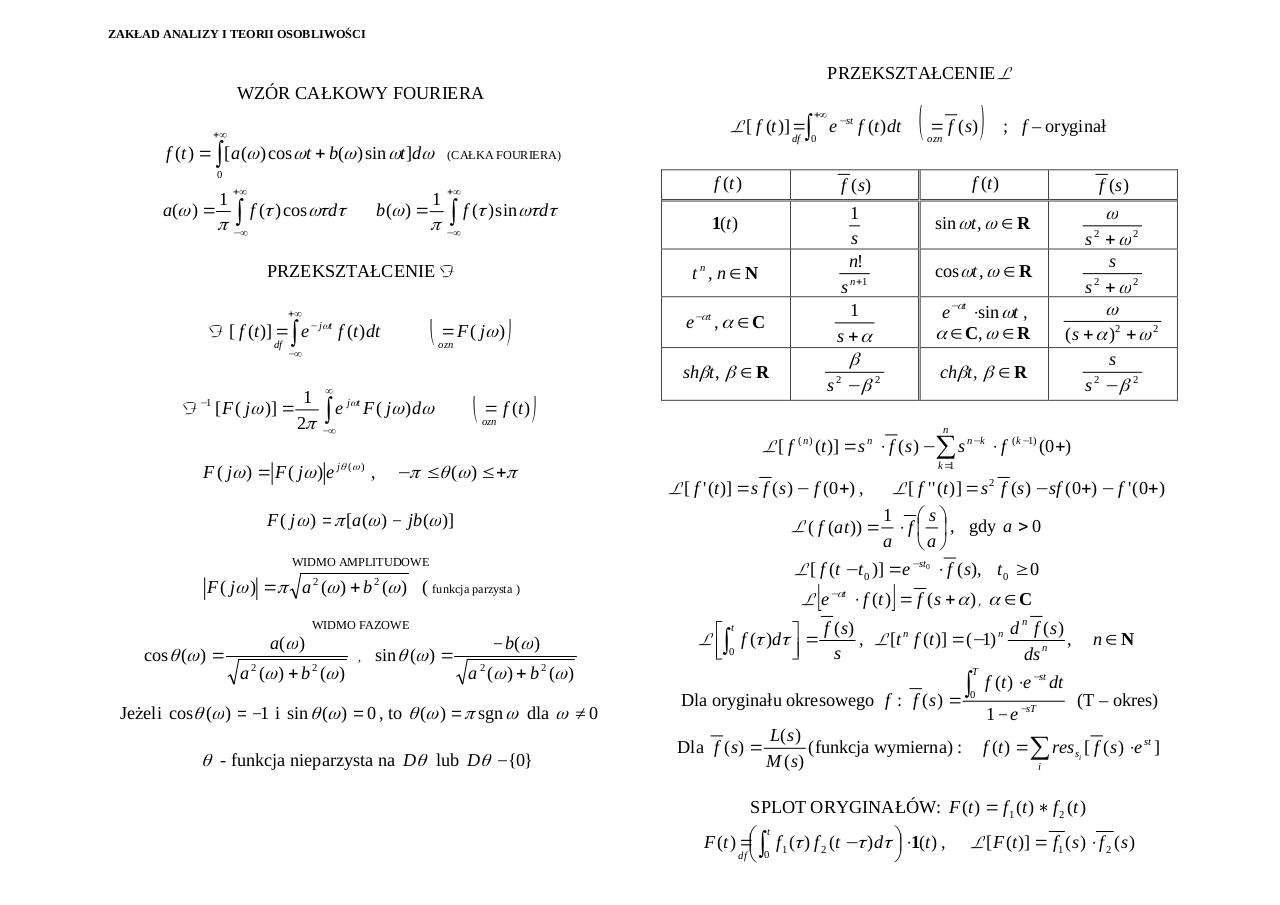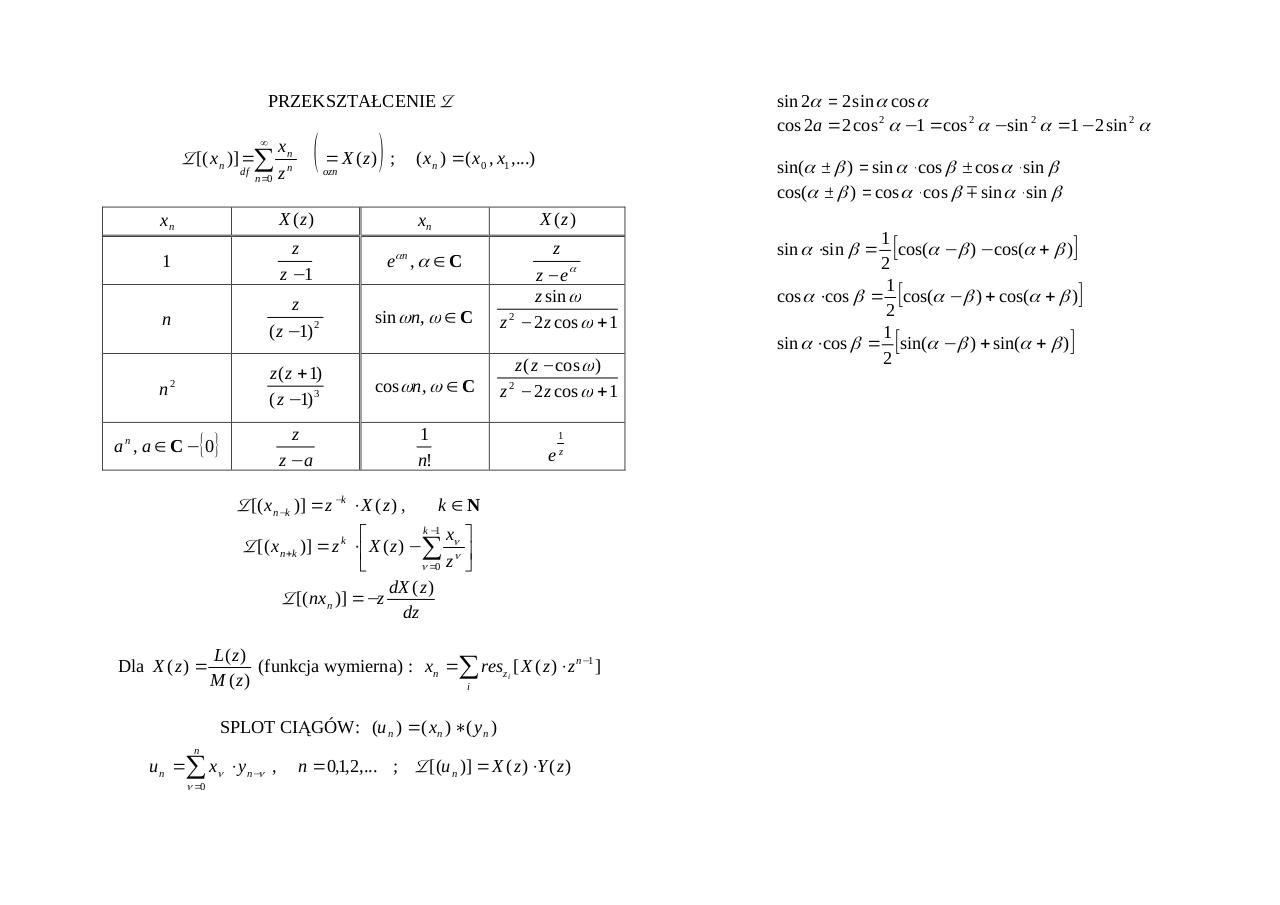# wzory .pdf

### File information

Original filename: wzory.pdf
Title: Tabelka z przeksztaÂ³ceniami caÂ³kowymi

This PDF 1.3 document has been generated by PDFCreator Version 0.9.0 / AFPL Ghostscript 8.53, and has been sent on pdf-archive.com on 04/05/2016 at 23:37, from IP address 188.146.x.x. The current document download page has been viewed 351 times.
File size: 44 KB (2 pages).
Privacy: public file

wzory.pdf (PDF, 44 KB)

### Document preview

PRZEKSZTAŁCENIE L
WZÓR CAŁKOWY FOURIERA
+∞

L [ f (t )] = ∫ e − st f (t )dt

+∞

df

f (t ) = ∫ [a(ω ) cos ωt + b(ω ) sin ωt ]dω
a (ω ) =

π

+∞

∫ f (τ ) cosωτdτ

1

b(ω ) =

π

−∞

f (t )

+∞

∫ f (τ ) sin ωτdτ

−∞

F [ f (t )] = ∫ e
df

− j ωt

( = F ( jω ) )

f (t )dt

1
F [ F ( jω )] =

∫e

j ωt

F ( j ω ) dω

−∞

e −αt , α ∈ C

( = f (t ))

− π ≤ θ (ω ) ≤ +π

a (ω )

a 2 (ω ) + b 2 (ω )

sin θ (ω ) =

− b(ω )
a 2 (ω ) + b 2 (ω )

JeŜeli cos θ (ω ) = −1 i sin θ (ω ) = 0 , to θ (ω ) = π sgn ω dla ω ≠ 0

θ - funkcja nieparzysta na Dθ lub Dθ − {0}

s +ω2
s
2
s +ω2

cos ωt , ω ∈ R

2

e −αt ⋅ sin ωt ,
α ∈ C, ω ∈ R

ω
(s + α )2 + ω 2

chβt , β ∈ R

s
s −β2
2

(n)

(t )] = s n ⋅ f ( s ) − ∑ s n− k ⋅ f ( k −1) (0+ )

L [ f ' (t )] = s f ( s ) − f (0+ ) ,

L [ f ' ' (t )] = s 2 f ( s ) − sf (0+ ) − f ' (0+ )
1 s
L ( f (at )) = ⋅ f   , gdy a &gt; 0
a a
L [ f (t − t 0 )] = e − st0 ⋅ f ( s ), t 0 ≥ 0

[

2

,

s −β
2

ω
2

k =1

F ( jω ) = π a (ω ) + b (ω ) ( funkcja parzysta )
cos θ (ω ) =

sin ωt , ω ∈ R

f (s )

n

WIDMO AMPLITUDOWE

WIDMO FAZOWE

1
s
n!

ozn

F ( jω ) = π [a (ω ) − jb(ω )]

2

f (t )

β

shβt , β ∈ R

L[ f

F ( jω ) = F ( jω ) e jθ (ω ) ,

; f – oryginał

ozn

f (s )

s n +1
1
s +α

ozn

−∞

−1

1(t )

tn, n∈N

PRZEKSZTAŁCENIE F
+∞

( = f (s))

(CAŁKA FOURIERA)

0

1

0

]

L e −αt ⋅ f (t ) = f ( s + α ) , α ∈ C
n
t
f (s)
n
n d f (s)

L ∫ f (τ )dτ =
, L [t f (t )] = (−1)
,
 0

s
ds n

T

Dla oryginału okresowego f :
Dla f ( s ) =

f ( s) =

L( s )
(funkcja wymierna) :
M (s)

0

f (t ) ⋅ e − st dt
1 − e − sT

n∈N
(T – okres)

f (t ) = ∑ res si [ f ( s ) ⋅ e st ]
i

SPLOT ORYGINAŁÓW: F (t ) = f 1 (t ) ∗ f 2 (t )
t
F (t ) = ∫ f 1 (τ ) f 2 (t − τ )dτ  ⋅ 1(t ) ,
df  0

L [ F (t )] = f 1 ( s ) ⋅ f 2 ( s )

sin 2α = 2 sin α cos α
cos 2a = 2 cos 2 α − 1 = cos 2 α − sin 2 α = 1 − 2 sin 2 α

PRZEKSZTAŁCENIE Z

Z [( x n )] = ∑
df

n =0

( = X ( z)) ;

xn
zn

ozn

( x n ) = ( x 0 , x1 ,...)

sin(α ± β ) = sin α ⋅ cos β ± cos α ⋅ sin β
cos(α ± β ) = cos α ⋅ cos β m sin α ⋅ sin β

xn

X (z )

xn

X (z )

1

z
z −1

e αn , α ∈ C

n

z
( z − 1) 2

sin ωn, ω ∈ C

z
z − eα
z sin ω
2
z − 2 z cos ω + 1

z ( z + 1)
( z − 1) 3

cos ωn, ω ∈ C

z ( z − cos ω )
z − 2 z cos ω + 1

z
z−a

1
n!

ez

n

2

a n , a ∈ C − {0}

Z [( x n −k )] = z − k ⋅ X ( z ) ,

2

1

k ∈N
k −1

x 

Z [( x n + k )] = z k ⋅  X ( z ) − ∑ νν 
ν =0 z 

dX ( z )
Z [(nx n )] = − z
dz
Dla X ( z ) =

L( z )
(funkcja wymierna) : xn = ∑ resz i [ X ( z ) ⋅ z n −1 ]
M ( z)
i
SPLOT CIĄGÓW: (u n ) = ( x n ) ∗ ( y n )

n

u n = ∑ xν ⋅ y n −ν ,
ν =0

n = 0,1,2,... ;

Z [(u n )] = X ( z ) ⋅ Y ( z )

1
[cos(α − β ) − cos(α + β )]
2
1
cos α ⋅ cos β = [cos(α − β ) + cos(α + β )]
2
1
sin α ⋅ cos β = [sin(α − β ) + sin(α + β )]
2
sin α ⋅ sin β =#### HTML Code

Copy the following HTML code to share your document on a Website or Blog

#### QR Code### Related keywords